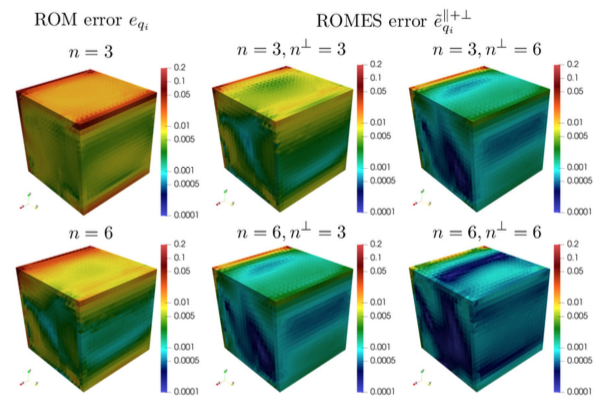# Statistical closure modeling for reduced-order models of stationary systems by the ROMES methodAn example of a full refinement.

### Abstract

This work proposes a technique for constructing a statistical closure model for reduced-order models (ROMs) applied to stationary systems modeled as parameterized systems of algebraic equations. The proposed technique extends the reduced-order-model error surrogates (ROMES) method to closure modeling. The original ROMES method applied Gaussian-process regression to construct a statistical model that maps cheaply computable error indicators (e.g., residual norm, dual-weighted residuals) to a random variable for either (1) the norm of the state error or (2) the error in a scalar-valued quantity of interest. Rather than target these two types of errors, this work proposes to construct a statistical model for the state error itself; it achieves this by constructing statistical models for the generalized coordinates characterizing both the in-plane error (i.e., the error in the trial subspace) and a low-dimensional approximation of the out-of-plane error. The former can be considered a statistical closure model, as it quantifies the error in the ROM generalized coordinates. Because any quantity of interest can be computed as a functional of the state, the proposed approach enables any quantity-of-interest error to be statistically quantified a posteriori, as the state-error model can be propagated through the associated quantity-of-interest functional. Numerical experiments performed on both linear and nonlinear stationary systems illustrate the ability of the technique (1) to improve (expected) ROM prediction accuracy by an order of magnitude, (2) to statistically quantify the error in arbitrary quantities of interest, and (3) to realize a more cost-effective methodology for reducing the error than a ROM-only approach in the case of nonlinear systems.

Publication
Submitted to the SIAM Journal on Uncertainty Quantification